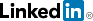cancel
Showing results for
Did you mean:
Frequent Visitor

## Avererage in column with variant data type

Hi all,

I am stuck in the matrix table in PowerBI.

See data below.

Column VALUE is formatted as numbers, TEXT as text and U_RPT_VALUE also as text.

 TEST_NUMBER NAME VALUE TEXT U_RPT_VALUE 721195 Component 1 0.1 0.1 < 0.2 721178 Component 1 0 0.0 < 0.2 721188 Component 2 4.33 4.33 4.33 721183 Component 3 0 0.00 < 5.9 721182 Component 3 0 0.00 < 5.9 721181 Component 3 0.56 0.56 < 5.9 721192 Component 4 0 Done Done 721198 Component 5 755 755 755 721199 Component 5 730 730 730

Now I want to have a matrix table with the average of each component.

The problem is that because the column contains data and text you can only choose count, first etc

This will give the incorrect data for Component 5.

So I created Measure = AVERAGE(Sheet2[VALUE]) but that will show incorrect values for component 3

I'm trying to build someting like:  If(U_RPT_VALUE contains < then show U_RPT_VALUE else (Measure = AVERAGE(Sheet2[VALUE]))

However, I cannot get it working due to the different data formats.

Do I make it myself to complicated and is there an easy way to accomplish this?

Thanks for the support!

Sander

1 ACCEPTED SOLUTION

Accepted SolutionsSuper User III

Hi @SanderB

Try the below

```Measure =
VAR _text = SELECTEDVALUE( 'Table'[U_RPT_VALUE] )
VAR _search = SEARCH( "<", _text, 1, 0 ) > 0
RETURN IF( _search, _text, AVERAGE( 'Table'[VALUE] ) )```

Best Regards,
Mariusz

If this post helps, then please consider Accepting it as the solution.

Please feel free to connect with me.8 REPLIES 8Super User III

Hi @SanderB

Try the below

```Measure =
VAR _text = SELECTEDVALUE( 'Table'[U_RPT_VALUE] )
VAR _search = SEARCH( "<", _text, 1, 0 ) > 0
RETURN IF( _search, _text, AVERAGE( 'Table'[VALUE] ) )```

Best Regards,
Mariusz

If this post helps, then please consider Accepting it as the solution.

Please feel free to connect with me.Frequent Visitor

Hi @Mariusz ,

Thank you very much, the use of a measure like that is totally new for me 🙂

It works perfect in the example, but on the complete database I get the "Expressions that yield variant data type" error.

This is caused by the Table[U_RPT_VALUE]

There is a lot of data in this table, dates, <,>,negative numbers,-, text and empty

Is there a way make this usefull? Otherwise I can create a new column and filter all the stuff out and see if it works

Frequent Visitor

It works! Corrected the column and it is perfect!

Thank you very much!

Frequent Visitor

Hi @Mariusz ,

Only one small question about AVERAGE.

Is it possible to have the decimal places dynamic?

I thought that the FIXED function will do this, but this does not work.

```Measure =
VAR _text = SELECTEDVALUE( RESULT[Merged] )
VAR _search = SEARCH( "<", _text, 1, 0 ) > 0
RETURN IF( _search, _text, FIXED(AVERAGE(RESULT[VALUE]),(RESULT[PLACES]),1))```

Thanks,

SanderSuper User III

HI @SanderB

You need some form of aggregation around RESULT[PLACES], try MIN or MAX like below.

```Measure =
VAR _text = SELECTEDVALUE( RESULT[Merged] )
VAR _search = SEARCH( "<", _text, 1, 0 ) > 0
RETURN IF( _search, _text, FIXED( AVERAGE( RESULT[VALUE] ), MAX( RESULT[PLACES] ), 1 ) )```

Frequent Visitor

Hi @Mariusz,

I looks like the FIXED function does not work there.

It will only give zero's and the filters are not working anymore, even without dynamic reference.

FIXED(AVERAGE( RESULT[VALUE] ),4,1 ))Super User III

Hi @SanderB

Can you give an example?

Frequent Visitor

Measure that is working with the incorrect decimals:

```Measure =
VAR _text = SELECTEDVALUE( RESULT[Merged] )
VAR _search = SEARCH( "<", _text, 1, 0 ) > 0
RETURN IF( _search, _text, AVERAGE( RESULT[VALUE] ))```Measure that is not working:

```Measure =
VAR _text = SELECTEDVALUE( RESULT[Merged] )
VAR _search = SEARCH( "<", _text, 1, 0 ) > 0
RETURN IF( _search, _text, FIXED( AVERAGE( RESULT[VALUE] ), MAX( RESULT[PLACES] ), 1 ) )```Values in table used

 Sample NAME VALUE PLACES U_RPT_VALUE Merged 1 Component1 0.02 2 < 0.05 <0.05 1 Component2 0.03 2 < 0.06 <0.06 1 Component3 5.29 2 5.29 5.29 1 Component4 5.35 2 5.35 5.35 1 Component5 5.3 2 5.30 5.3 1 Component6 1.0208 4 1.0208 1.0208

Thanks!

SanderAnnouncements#### Power Platform Community Conference

Check out the on demand sessions that are available now!#### Create an end-to-end data and analytics solution

Learn how Power BI works with the latest Azure data and analytics innovations at the digital event with Microsoft CEO Satya Nadella.Top Solution Authors
Top Kudoed Authors
Users online (1,537)